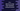# C++ program to return an array from a function using a structure## C++ program to return an array from a function using a structure:

In this post, we will learn how to return an array from a function by using a structure. If you want to return an array from a function in C++, you can use a pointer or you can define a structure that includes an array.

Let’s try it with different examples.

### C++ program to return an array using a structure:

Let’s take a look at the below example:

``````#include <iostream>
using namespace std;

struct arrayholder
{
int odd;
};

arrayholder getArray()
{
arrayholder a;

for (int i = 0; i < 10; i++)
{
if (i % 2 != 0)
{
a.odd[i] = i;
}
else
{
a.odd[i] = -1;
}
}

return a;
}

int main()
{
arrayholder arr = getArray();

for (int i = 0; i < 10; i++)
{
cout << arr.odd[i] << ' ';
}
cout << endl;
return 0;
}``````

Here,

• arrayholder is a structure with an array of 10 elements, odd.
• getArray method returns one arrayholder.
• Inside getArray, we are creating one arrayholder variable a and running one for loop. This loop runs from 0 to 10 and adds all odd values to the odd positions. For the even positions, it adds -1.

It will print the below output:

``-1 1 -1 3 -1 5 -1 7 -1 9 ``

## Returning multiple arrays:

The advantage of this method is that we can return more than 1 arrays using a structure. For example:

``````#include <iostream>
using namespace std;

struct arrayholder
{
int odd;
int even;
};

arrayholder getArray()
{
arrayholder a;

for (int i = 0; i < 10; i++)
{
if (i % 2 != 0)
{
a.odd[i] = i;
a.even[i] = -1;
}
else
{
a.odd[i] = -1;
a.even[i] = i;
}
}

return a;
}

int main()
{
arrayholder arr = getArray();

for (int i = 0; i < 10; i++)
{
cout << arr.odd[i] << ' ';
}
cout << endl;

for (int i = 0; i < 10; i++)
{
cout << arr.even[i] << ' ';
}
cout << endl;
return 0;
}``````

The structure holds two arrays: odd and even. If you run this program, it will print the below output:

``````-1 1 -1 3 -1 5 -1 7 -1 9
0 -1 2 -1 4 -1 6 -1 8 -1 ``````